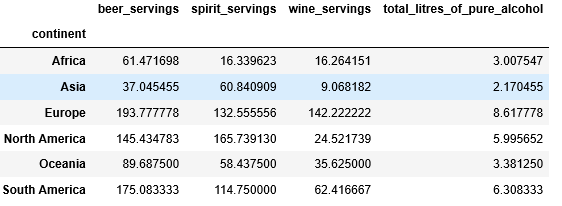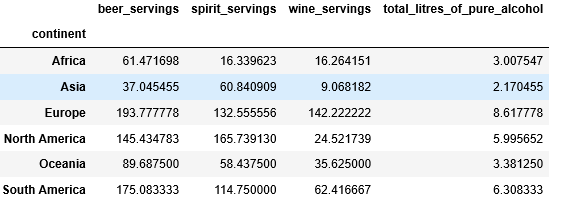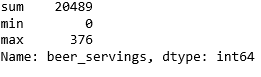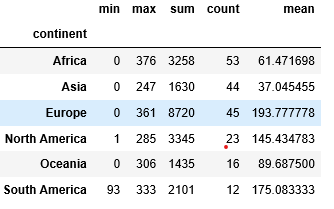# How to combine Groupby and Multiple Aggregate Functions in Pandas?

• Difficulty Level : Easy
• Last Updated : 10 May, 2020

Pandas is a Python package that offers various data structures and operations for manipulating numerical data and time series. It is mainly popular for importing and analyzing data much easier. It is an open-source library that is built on top of NumPy library.

### Groupby()

Pandas `dataframe.groupby()` function is used to split the data in dataframe into groups based on a given condition.

Example 1:

 `# import library ` `import` `pandas as pd ` ` `  `# import csv file ` `df ``=` `pd.read_csv(``"https://bit.ly/drinksbycountry"``) ` ` `  `df.head() `

Output:Example 2:

 `# Find the average of each continent ` `# by grouping the data   ` `# based on the "continent". ` `df.groupby([``"continent"``]).mean() `

Output:### Aggregate()

Pandas `dataframe.agg()` function is used to do one or more operations on data based on specified axis

Example:

 `# here sum, minimum and maximum of column  ` `# beer_servings is calculatad ` `df.beer_servings.agg([``"sum"``, ``"min"``, ``"max"``]) `

Output:Using These two functions together: We can find multiple aggregation functions of a particular column grouped by another column.

Example:

 `# find an aggregation of column "beer_servings" ` `# by grouping the "continent" column. ` `df.groupby(df[``"continent"``]).beer_servings.agg([``"min"``, ` `                                               ``"max"``, ` `                                               ``"sum"``, ` `                                               ``"count"``, ` `                                               ``"mean"``]) `

Output:My Personal Notes arrow_drop_up
Recommended Articles
Page :